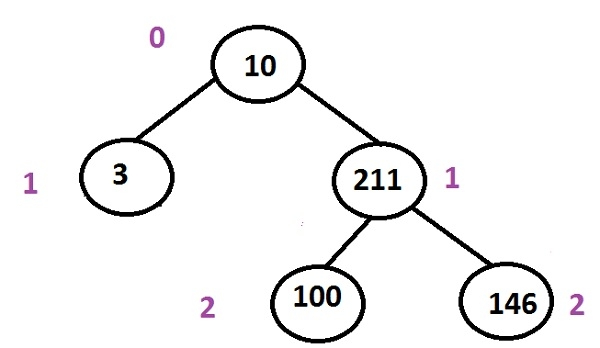# Print the first shortest root to leaf path in a Binary Tree in C++ Programming.

Given the binary tree the program must find out the shortest path from the root to leaf amongst many given paths.

Since we traverse the tree from left to right, so if there are multiple shortest paths from the root to leaf than the program will print the first traversed the shortest path on the left side of a tree.

We can use a queue that will traverse each level using Level order traversal and the path with the least number of levels will be printed as it will be the shortest path from the root to leafIn the above tree, multiple paths from the root to leaf are

10 -> 3 (this one is the shortest path amongst all)
10 -> 211 -> 100
10 -> 211 -> 146

## Example

Input  : 10 3 211 100 146
Output : 10 3

## Algorithm

Step 1 -> create a structure of a node as
struct node
struct node *left, *right
int data
End
Step 2 -> function to create a node
node* newnode(int data)
node *temp = new node
temp->data = data
temp->left = temp->right= NULL
return temp
Step 3 -> create function for calculating path
void path(int data, unordered_map <int,int> prnt)
IF prnt[data] = data
Return
End
path(prnt[data], prnt)
print prnt[data]
step 4 -> function for finding out the left path
void left(Node* root)
create STL queue<Node*> que
que.push(root)
int leaf = -1
Node* temp = NULL
Create STL unordered_map<int, int> prnt
prnt[root->data] = root->data
Loop While !que.empty()
temp = que.front()
que.pop()
IF !temp->left && !temp->right
leaf = temp->data
break
End
Else
IF temp->left
que.push(temp->left)
prnt[temp->left->data] = temp->data
End
IF temp->right
que.push(temp->right)
prnt[temp->right->data] = temp->data
End
End
End
path(leaf, prnt)
print leaf
Step 5 -> In main()
Create tree using Node* root = newnode(90)
root->left = newnode(21)
call left(root)
stop

## Example

Live Demo

#include <bits/stdc++.h>
using namespace std;
// structure of a node
struct Node {
struct Node *left,*right;
int data;
};
//function to create a new node
Node* newnode(int data){
Node* temp = new Node;
temp->data = data;
temp->left = NULL;
temp->right = NULL;
return temp;
}
//function to set a path
void path(int data, unordered_map <int,int> prnt) {
if (prnt[data] == data)
return;
path(prnt[data], prnt);
cout << prnt[data] << " ";
}
//function for a leaf path
void left(Node* root) {
queue<Node*> que;
que.push(root);
int leaf = -1;
Node* temp = NULL;
unordered_map<int, int> prnt;
prnt[root->data] = root->data;
while (!que.empty()){
temp = que.front();
que.pop();
if (!temp->left && !temp->right{
leaf = temp->data;
break;
} else {
if (temp->left){
que.push(temp->left);
prnt[temp->left->data] = temp->data;
}
if (temp->right){
que.push(temp->right);
prnt[temp->right->data] = temp->data;
}
}
}
path(leaf, prnt);
cout << leaf << " ";
}
int main(){
Node* root = newnode(90);
root->left = newnode(21);
root->right = newnode(32);
root->left->left = newnode(45);
root->right->left = newnode(52);
root->right->right = newnode(27);
root->left->left->left = newnode(109);
root->left->left->right = newnode(101);
root->right->right->left = newnode(78);
left(root);
return 0;
}

## Output

if we run the above program then it will generate the following output

90 32 52